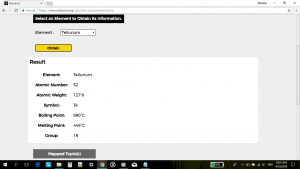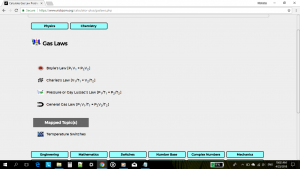# The Calculator For Chemists

Nickzom Calculator+ is a 100% all in one package that solves over 32,000 calculations and shows the steps of the calculations. One of the sections it handles is Chemistry.
Nickzom Calculator+ solves over 8,500+ calculations in Chemistry and shows the steps, formulas, parameters and answer accurately and comprehensively.

Nickzom Calculator+ handles the following major chapters of Chemistry:

– Basic Chemistry
– Kinetic Theory of Gases
– Ideal Gas Equation
– Graham’s Law of Diffusion
– Dilution Equation
– Solution Purity
– Empirical Formula
– Yield
– Force | Coulomb’s Law
– De Broglie’s Law
– Density
– Number of Particles in a Substance
– Linear Momentum
– Mass
– Percentage of an Element
– Number Concentration
– Volume Concentration
– Molar Concentration
– Mass Concentration
– Number of Moles
– Molar Mass
– Molarity
– Normality
– Heat
– Electrolysis
– Kinetic Energy per Molecule
– Relationship between Energy and Principal Quantum Number
– Kinetic Energy per Mole
– Osmotic Pressure
– Freezing Point Depression
– Boiling Point Elevation
– Elements
– Gas Laws
– Boyle’s Law
– Charles’ Law
– Pressure or Gay Lussac’s Law
– General Gas Law

Within these major topics there are sub topics right up to selections of the various parameters you desire to find.

To obtain this calculator via web

Visit https://www.nickzom.org/calculator
Scroll to the Bottom
Click on Access Our Professional Version
Register with Us
Make a Payment of NGN 1,500
Select Chemistry in the Drop Down
Click on “Open Section”

To obtain this calculator via Google Play Store (For Android)